# Area Of A Quadrilateral - Definition with Examples

The Complete K-5 Math Learning Program Built for Your Child

• 40 Million Kids

Loved by kids and parent worldwide

• 50,000 Schools

Trusted by teachers across schools

• Comprehensive Curriculum

Aligned to Common Core

## What is Area of a Quadrilateral?

A quadrilateral is a polygon we obtain by joining four vertices, and it has four sides and four angles. There are two types of quadrilaterals⁠ — regular and irregular quadrilaterals. Some examples of the quadrilaterals are square, rectangle, rhombus, trapezium, and parallelogram.

The area of a polygon refers to the space occupied by the flat shape. It is the combined sum of the area of the regular and irregular triangles within.

## Measuring the area of a quadrilateral

To evaluate the area of a quadrilateral, we divide it into two basic geometric figures, such as triangles. Then we find the area of the two individual triangles using the formula and add these areas to find the area of the quadrilateral.

## Calculating the area of a quadrilateral

• Draw a diagonal AC connecting two opposite vertices of the quadrilateral ABCD.

• Draw a perpendicular each from the other two vertices

(B and D) on the diagonal AC.

• The area of the quadrilateral will be:

Area of quadrilateral ABCD = Area of △ABC + Area of △ADC

So, area of quadrilateral ABCD = (½ × AC × BE) + (½ × AC × DF)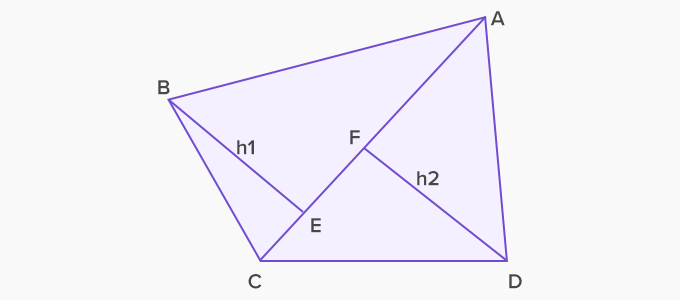We can calculate the area of different types of quadrilaterals by using the given formula. For the quadrilateral ABCD, if we use centimeter as the unit of measurement, the unit of measure for the area will be cm2 .

## Area of a parallelogram

To evaluate the area of a parallelogram, draw a perpendicular from one of the vertices to the base. This perpendicular is the height. Thus, the area will be the product of base and height.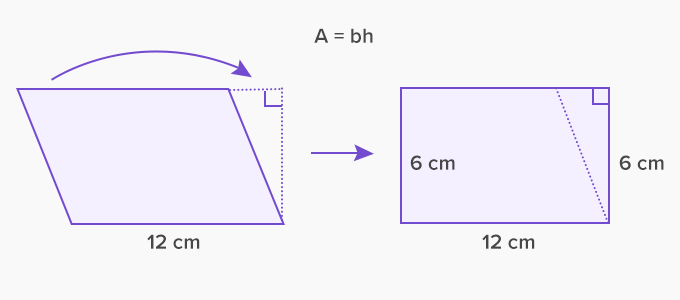Area of parallelogram = base x height

Area = 12 × 6 = 72 cm

## Area of a rhombus

To find the area of a rhombus, we divide the quadrilateral into two equal isosceles triangles using the two diagonals. In the given rhombus ABCD, the point of intersection of these diagonals is E. Thus the area of the rhombus is: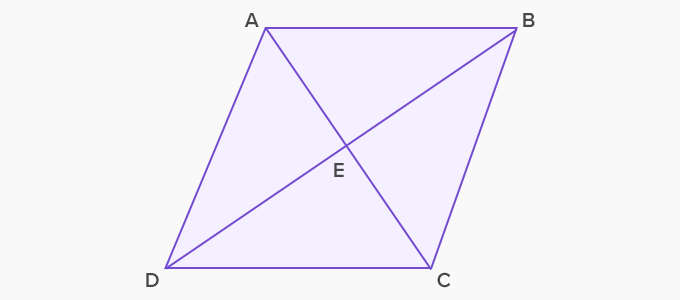Area of rhombus ABCD = Area of △ABC + Area of △ADC

⟹  Area of rhombus ABCD = (½ x AC x BE) + (½ x AC x ED)

⟹  Area of rhombus ABCD  = ½ x AC (BE + ED)

⟹  Area of rhombus ABCD = ½ x AC x BD

## Area of a square

Using this relationship we can also find the area of a square ABCD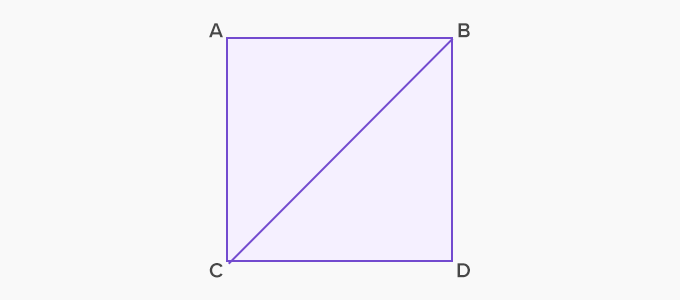Area of square ABCD = Area of △ABC  + Area of △BCD

⟹ Area of △ABC  = ½ * AC * AB

⟹ Area of △ABC  = ½ * AC * AC (as AC = AB)

⟹ Area of △ABC  = ½ * AC2

Similarly, Area of △BCD  = ½ * CD2

Since, AC = CD, Area of △BCD  will be ½ * AC2

Thus, area of square ABCD  = 2 * (½ * AC2) = AC2

Hence, Area of square ABCD is the square of the side.

## Area of a rectangle

The area of a rectangle using the above formula will yield the product of its two adjacent sides, base and height. We represent it as: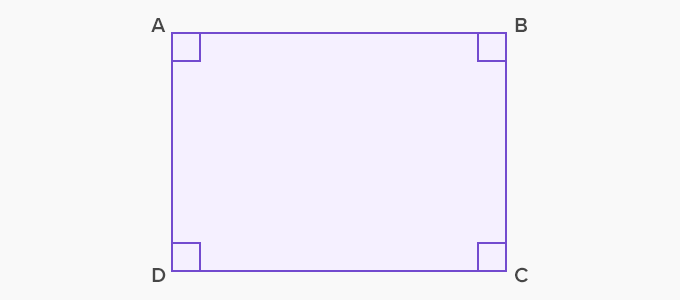• Area (ABCD) = AB x BC

## Application

The real-life application of quadrilaterals and its area are highly useful in the fields of design, agriculture, and architecture. The concept is highly useful in the advanced designing of navigation maps scaled to actual distances and areas with precision.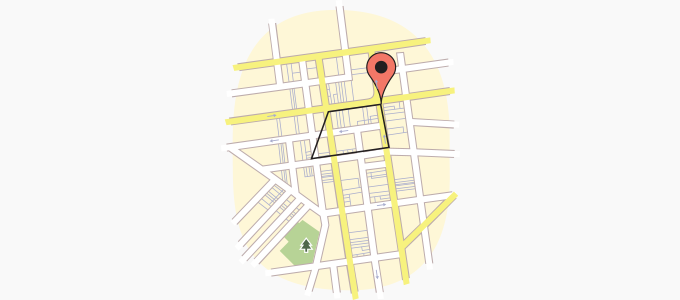The area covered by a quadrilateral formed by joining four different places on a map

 Fun Facts The term quadrilateral is a combination of words Quadri + Lateral which means “four sides”. Except for square, all the quadrilaterals are irregular quadrilaterals. They are also known as “Quadrangle” and “Tetragon” (four and a polygon). The sum of all the angles within a quadrilateral is always 360°.

### Related math vocabulary

Won Numerous Awards & Honors